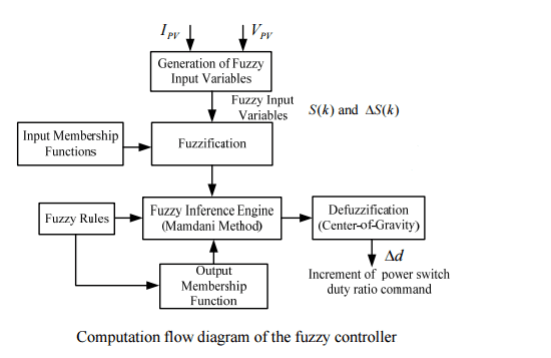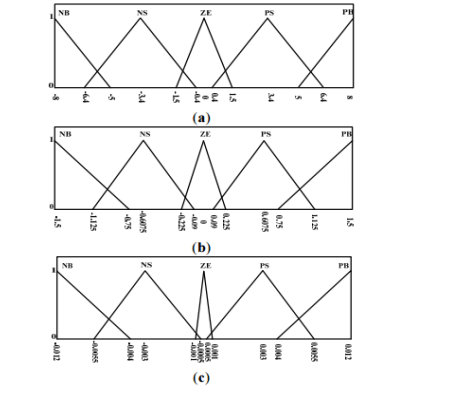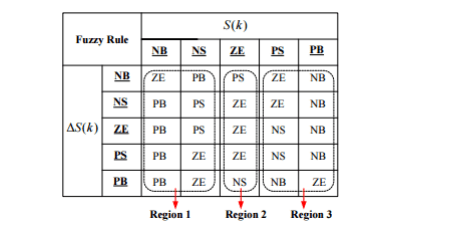Maximum power point tracker

EXERCISE: MAXIMUM POWER POINT TRACKER FOR A PHOTOVOLTAIC SYSTEM

Maximum Power Point Tracking (MPPT), or MPP controller or MPP tracker is a principle to follow the maximum power point of a nonlinear electrical generator. MPPT systems are generally associated with PV or wind generators. In this case, we propose to develop a fuzzy MPPT controller for a photovoltaic system (figure 1).Figure 1: Solar power maximum power point tracking system.

The principle of this controller is to provoke perturbation by acting (decrease or increase) on the PWM duty cycle D and observing the effect on the output PV power. If the instant power Ppv(k) is greater than the previous computed power Ppv(k-1), then the direction of perturbation is maintained otherwise it is reversed. Referring to figure 2  this can be detailed as follows:

– when dPpv/dVpv > 0 , the voltage is increased, this is done through D(k) = D(k-1) – C, ( C : incrementation step),
– when dPpv/dVpv < 0, the voltage is decreased through D(k) = D(k-1) + C.Figure 2: Power-voltage characteristic of a PV module.

The fuzzy logic MPPT system uses the slope of the PV cell’s Power-Voltage (P-V) curve (S(k)) and variation of slope ( ∆S(k) ) as the fuzzy input variables. These variables are defined using the following equations:A five-term fuzzy set, positive big (pB), positive small (pS), zero (zE), negative small (nS), and negative big (nB), is defined to describe each linguistic variable. Output from the fuzzy controller (duty ratio command of the buck-boost converter) would change the output voltage and current of the PV cell.  As Vout =( D/1-D)×Vpv , if we increase D, we decrease Vpv. So for example, if S(k) is negative and ∆S(k) is negative than we have to decrease Vpv so to increase D.

We propose to develop a fuzzy MPPT controller for the photovoltaic system.
1) Make a computation flow diagram (“organigramme”) of the fuzzy controller.
2) Draw inputs and output membership functions with notations (nB, nS, pB, pS, zE).
Input 1 (S(k)) range: [-8 8]
Input 2 (∆S(k)) range: [- 1.5 1.5]
Output (∆D: duty cycle variation) range: [-0.012 0.012].
3) Cite at least five if then rules as example.
4) Give the fuzzy logic rules table (Fuzzy Associative Matrix)

CORRECTION

1)2)Membership function for Algorithm (i):

(a) Membership function for P-V slope;

(b) Membership function for changes of slope;

(c) Membership function for increment of duty ratio command.

3) If S(k) is nB and ∆S(k) is nB then ∆D is zE
If S(k) is nB and ∆S(k) is nS then ∆D is pB
If S(k) is nS and ∆S(k) is nS then ∆D is pS
If S(k) is zE and ∆S(k) is zE then ∆D is zE
If S(k) is pB and ∆S(k) is pB then ∆D is zE

4)